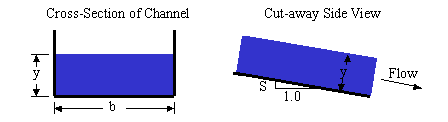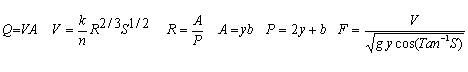Rectangular Open Channel Design Calculation

Rectangular open channel for river or laboratory hydraulics - Discharge, water depth, slope, velocity, Froude

Register to enable "Calculate" button.

 Select calculation, units: © 2015 LMNO Engineering, Enter n, S, y, b Enter Q, n, S, y Enter Q, n, S, b Enter Q, n, S, y/b Enter Q, n, y, b Enter Q, S, y, b Enter V, n, S, y Enter V, n, S, b Enter V, n, S, y/b Enter V, n, y, b Enter V, S, y, b Research, and Software, Ltd. www.LMNOeng.com Discharge, Q: m3/s ft3/s or cfs gal/min (U.S.) gal/day (U.S.) Acre-ft/yr Velocity, V: m/s ft/s Water Depth, y: m cm ft inch Width, b: m cm ft inch Ratio y/b: Manning Roughness, n: Channel Slope, S (m/m): Area, A: m2 ft2 Wetted Perimeter, P: m ft Hydraulic Radius, R: m Froude Number, F:Units: cfs=cubic foot per second, cm=centimeter, ft=foot, gal=U.S. gallon, m=meter, min=minute, s=second, yr=year

Introduction
The equation beginning V=.... is called the Manning Equation. It is a semi-empirical equation and is the most commonly used equation for uniform steady state flow of water in open channels (see Discussion and References for Open Channel Flow for further discussion). Because it is empirical, the Manning equation has inconsistent units which are handled through the conversion factor k. Uniform means that the water surface has the same slope as the channel bottom. Uniform flow is actually only achieved in channels that are long and have an unchanging cross-section. However, the Manning equation is used in other situations despite not strictly achieving these conditions.

To model a channel that has sloped sides, rather than vertical sides, please see our trapezoidal open channel calculator. We wrote the rectangular open channel hydraulics calculator before we wrote the trapezoidal channel calculator. Even though the trapezoidal calculator solves the case of a rectangular channel, we have kept the rectangular open channel flow calculator on the website.

In our rectangular open channel flow calculation, most of the combinations of inputs have analytic (closed form) solutions to compute the unknown variables; however, two require numerical solutions ("Enter Q, n, S, y" and "Enter Q, n, S, b"). Our numerical solutions utilize a cubic solver. All of our calculations utilize double precision.

Variables [L]=Length units, [T]=Time units.                     To top of page
A = Flow cross-sectional area, determined normal (perpendicular) to the bottom surface [L2].
b = Channel bottom width [L].
F = Froude number. F is a non-dimensional parameter indicating the relative effect of inertial effects to gravity effects. Flows with F<1 are low velocity flows called subcritical. F>1 are high velocity flows called supercritical. Subcritical flows are controlled by downstream obstructions while supercritical flows are affected by upstream controls. F=1 flows are called critical.
g = acceleration due to gravity = 32.174 ft/s2 = 9.8066 m/s2. g is used in the equation for Froude number.
k = unit conversion factor = 1.49 if English units = 1.0 if metric units. Our software converts all inputs to SI units (meters and seconds), performs the computations using k=1.0, then converts the computed quantities to units specified by the user. Required since the Manning equation is empirical and its units are inconsistent.
n = Manning coefficient. n is a function of the channel material, such as gravel, earthy, weedy, concrete, etc. Values for n can be found in the table below of Manning's n coefficients.
P = Wetted perimeter [L]. P is the contact length (in the cross-section) between the water and the channel.
Q = Discharge or flow rate [L3/T].
R = Hydraulic radius of the flow cross-section [L].
S = Slope of channel bottom or water surface [L/L]. Vertical distance divided by horizontal distance.
V = Average velocity of the water [L/T].
y = Water depth measured normal (perpendicular) to the bottom of the channel [L]. If the channel has a small slope (S), then entering the vertical depth introduces only minimal error.

Manning's n Coefficients                      To top of page
The table shows the Manning n values for materials that might be used in open channels. These values were compiled from the references listed under Discussion and References and in the references at the bottom of this web page (note the footnotes which refer to specific references).

 Material Manning n Material Manning n Natural Streams Excavated Earth Channels Clean and Straight 0.030 Clean 0.022 Major Rivers 0.035 Gravelly 0.025 Sluggish with Deep Pools 0.040 Weedy 0.030 Stony, Cobbles 0.035 Metals Floodplains Brass 0.011 Pasture, Farmland 0.035 Cast Iron 0.013 Light Brush 0.050 Smooth Steel 0.012 Heavy Brush 0.075 Corrugated Metal 0.022 Trees 0.15 Non-Metals Glass 0.010 Finished Concrete 0.012 Clay Tile 0.014 Unfinished Concrete 0.014 Brickwork 0.015 Gravel 0.029 Asphalt 0.016 Earth 0.025 Masonry 0.025 Planed Wood 0.012 Unplaned Wood 0.013 Corrugated Polyethylene (PE) with smooth inner walls a,b 0.009-0.015 Corrugated Polyethylene (PE) with corrugated inner walls c 0.018-0.025 Polyvinyl Chloride (PVC) with smooth inner walls d,e 0.009-0.011

Error Messages                      To top of page
"An input is ≤ 0".  All inputs must be positive.

"Infeasible input. Output < 0".  One or more computed values are negative, which is physically impossible.

References             To top of page
Footnotes refer to Manning n table above. All equations and other Manning n values were obtained from the references listed in our Discussion and References page.

a Barfuss, Steven and J. Paul Tullis. Friction factor test on high density polyethylene pipe. Hydraulics Report No. 208. Utah Water Research Laboratory, Utah State University. Logan, Utah. 1988.

c Barfuss, Steven and J. Paul Tullis. Friction factor test on high density polyethylene pipe. Hydraulics Report No. 208. Utah Water Research Laboratory, Utah State University. Logan, Utah. 1994.

e Bishop, R.R. and R.W. Jeppson. Hydraulic characteristics of PVC sewer pipe in sanitary sewers. Utah State University. Logan, Utah. September 1975.

d Neale, L.C. and R.E. Price. Flow characteristics of PVC sewer pipe. Journal of the Sanitary Engineering Division, Div. Proc 90SA3, ASCE. pp. 109-129. 1964.

b Tullis, J. Paul, R.K. Watkins, and S. L. Barfuss. Innovative new drainage pipe. Proceedings of the International Conference on Pipeline Design and Installation, ASCE. March 25-27, 1990.

LMNO Engineering, Research, and Software, Ltd.
7860 Angel Ridge Rd.   Athens, Ohio 45701  USA   Phone: (740) 592-1890
LMNO@LMNOeng.com    http://www.LMNOeng.com

To: